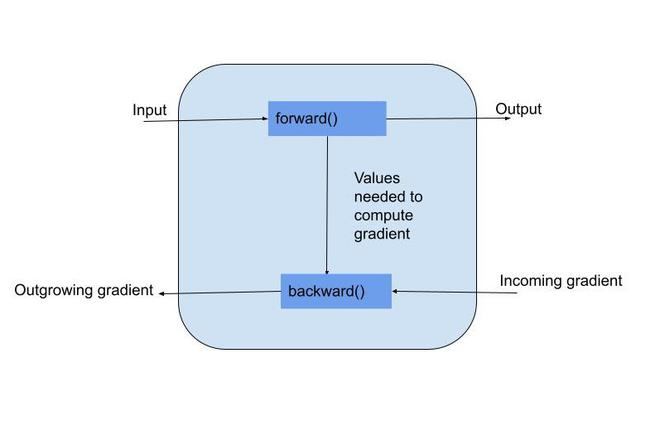GeeksforGeeks App
Open AppBrowser
Continue

## Related Articles

PyTorch is a python library developed by Facebook to run and train the machine and deep learning algorithms. In a neural network, we have to perform backpropagation which involves optimizing the parameter to minimize the error in its prediction.

For this PyTorch offers torch.autograd that does automatic differentiation by collecting all gradients. Autograd does this by keeping a record of data(tensors) & all executed operations in a directed acyclic graph consisting of function objects. In this DAG, leaves are the input tensors, roots are the output tensors. By tracing this graph from roots to leaves we can automatically compute the gradients using the chain rule.A variable is an automatic differentiation tool given a forward formulation. It wraps a variable. Variable supports nearly all the APIs defined by a Tensor. While defining a variable we pass the parameter requires_grad which indicates if the variable is trainable or not. By default, it is set to false. An example is depicted below to understand it more clearly.

Example 1:

## Python3

 `# importing libraries``import` `torch``from` `torch.autograd ``import` `Variable`` ` `# packing the tensors with Variable``a ``=` `Variable(torch.tensor([``5.``, ``4.``]), requires_grad``=``True``)``b ``=` `Variable(torch.tensor([``6.``, ``8.``]))`` ` `# polynomial function with a,b as variable``y ``=` `((a``*``*``2``)``+``(``5``*``b))``z ``=` `y.mean()``print``(``'Z value is:'``, z)`

Output:

Thus, in the above forward pass, we compute a resulting tensor maintaining the gradient function in DAG. After that when backward is called, it follows backward with the links created in the graph to backpropagate the gradient and accumulates them in the respective variable’s grad attribute.

Example 2:

## Python3

 `# importing libraries``import` `torch``from` `torch.autograd ``import` `Variable`` ` `# packing the tensors with Variable``a ``=` `Variable(torch.tensor([``5.``, ``4.``]), requires_grad``=``True``)``b ``=` `Variable(torch.tensor([``6.``, ``8.``]))`` ` `# polynomial function with a,b as variable``y ``=` `((a``*``*``2``)``+``(``5``*``b))``z ``=` `y.mean()`` ` `# dy/da=2*a=10,8``# dy/db=5`` ` `# computing gradient``z.backward()`` ` `# printing out``print``(``'Gradient of a'``, a.grad)``print``(``'Gradient of b'``, b.grad)`

Output:

Above you can notice that b’s gradient is not updated as in this variable requires_grad is not set to true. This is where Autograd comes into the picture.

Autograd is a PyTorch package for the differentiation for all operations on Tensors. It performs the backpropagation starting from a variable. In deep learning, this variable often holds the value of the cost function. Backward executes the backward pass and computes all the backpropagation gradients automatically.

First, we create some random features, e.g., weight and bias vectors. Perform a linear regression by multiplying x, W matrices. Then calculate the squared error and call the backward function to backpropagate, updating the gradient value of each variable. At last, we optimize the weight matrix and print it out.

Example:

## Python3

 `# importing libraries``import` `torch``from` `torch.autograd ``import` `Variable`` ` `# creating random tensors for weights and wrap them in variables``x ``=` `Variable(torch.randn(``1``, ``10``), requires_grad``=``False``)``W ``=` `Variable(torch.randn(``10``, ``1``), requires_grad``=``True``)  ``# weight matrix``b ``=` `Variable(torch.randn(``1``), requires_grad``=``True``)  ``# bias vector``y ``=` `Variable(torch.tensor([[``0.822``]]))`` ` `# performing matrix multiplication to compute output``y_pred ``=` `torch.matmul(x, W)``+``b`` ` `# calculating loss``loss ``=` `(y_pred``-``y).``pow``(``2``)``print``(loss)`` ` `# computing gradient``loss.backward()`` ` `print``(W.grad)``print``(b.grad)`` ` `lr ``=` `0.001`` ` `# updating the weight matrix after backpropagation``with torch.no_grad():``    ``W ``=` `W``-``(lr``*``W.grad.data)``print``(W)`

Output:

```tensor([[1.3523]], grad_fn=<PowBackward0>)
tensor([[-0.4488],
[ 1.8151],
[ 3.5312],
[ 1.4467],
[ 2.8628],
[-0.9358],
[-2.7980],
[ 0.2670],
[-0.0399],
[ 0.1995]])
tensor([2.3258])
tensor([[ 1.1908],
[ 0.0301],
[-0.2003],
[ 0.6922],
[ 2.1972],
[ 0.0633],
[ 0.7101],
[-0.5169],
[ 0.7412],
[ 0.7068]])```

My Personal Notes arrow_drop_up